# Smart Shoe Sensor

Insole for a shoe with sensors that measure weight applied on different areas of the foot and generates a colormap based on that data.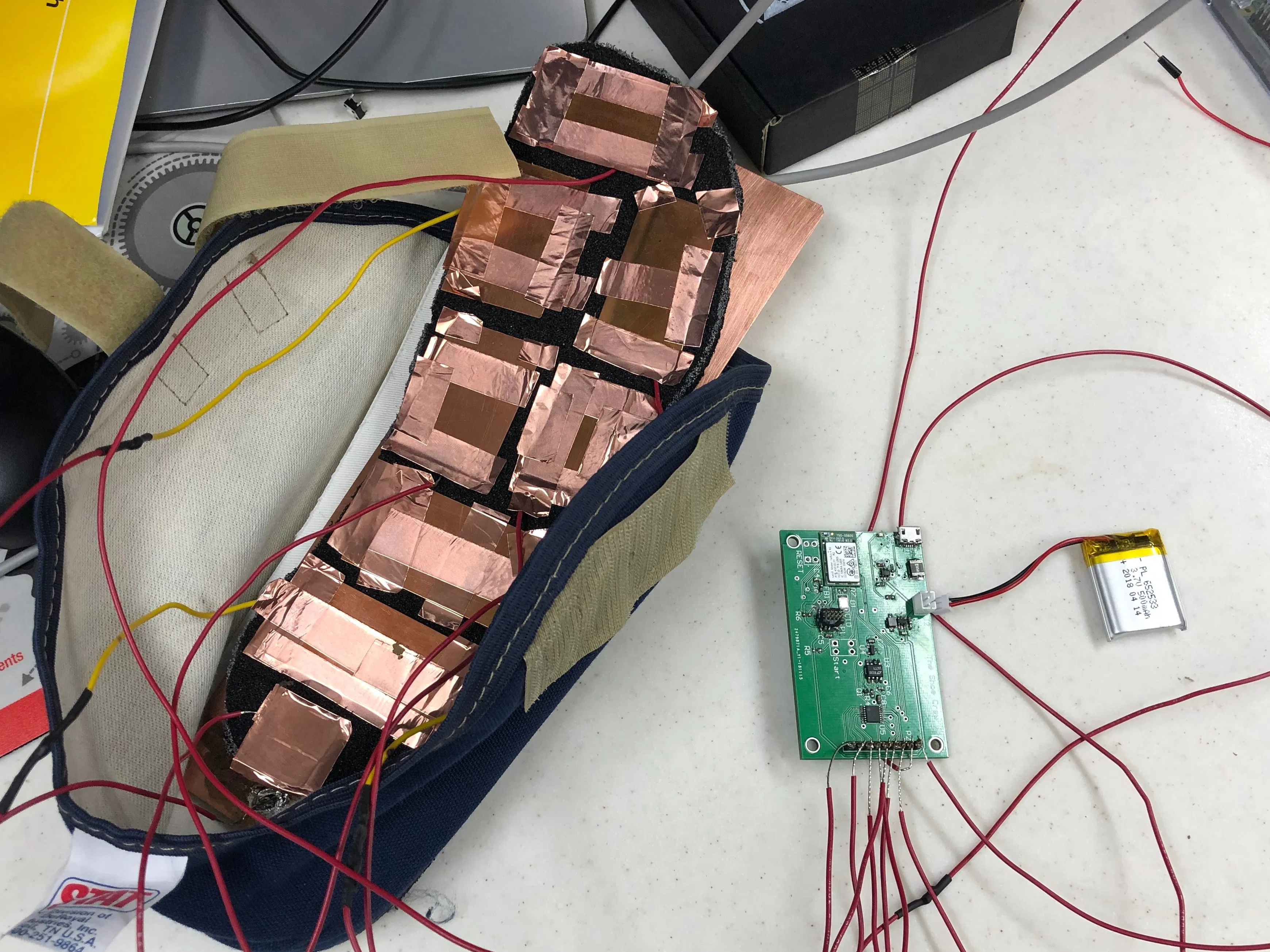## Things used in this project

### Hardware components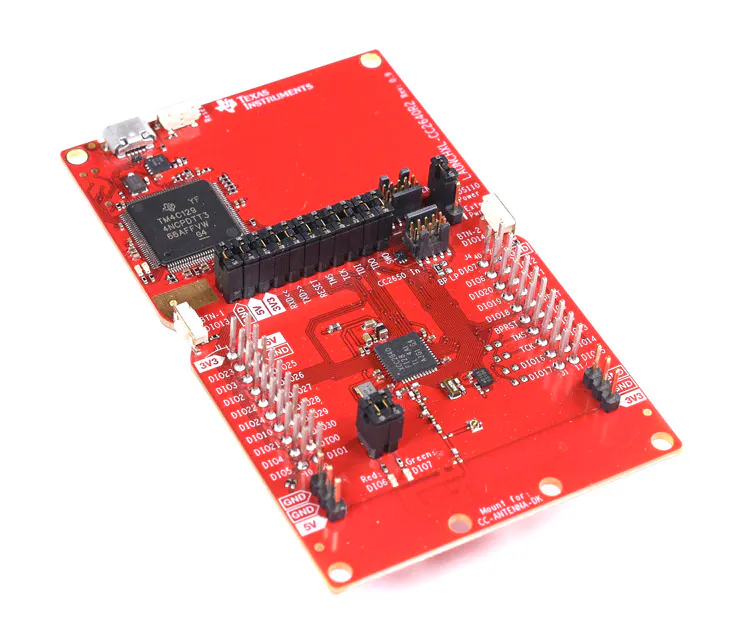×1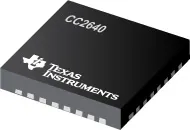Texas Instruments CC2640
×1
 SaBLE-x-R2 Bluetooth Low Energy (BLE) Module
×1Raspberry Pi 3 Model B
×1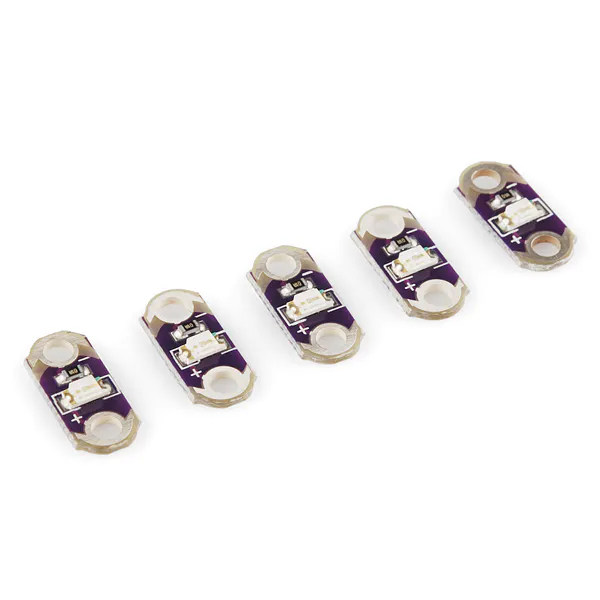×1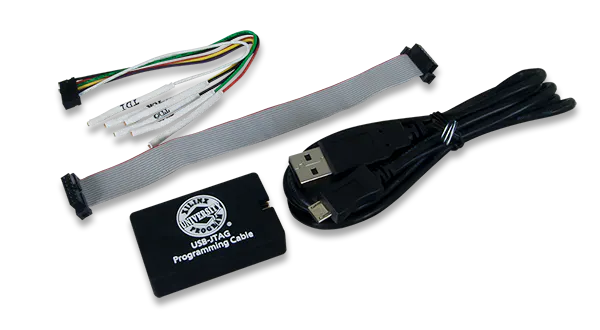Xilinx XUP USB-JTAG Programming Cable
×1
 3.7V Lithium Ion Battery
×1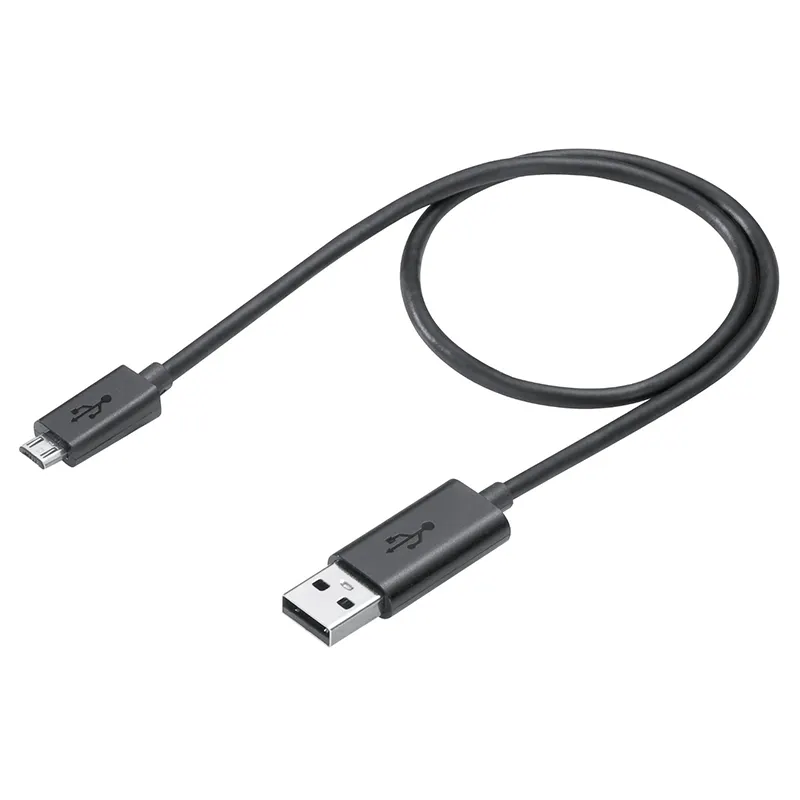Micro-USB to USB Cable (Generic)
×1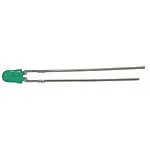3 mm LED: Green
×1
 Texas Instruments BQ21040DBVT Charger IC
×1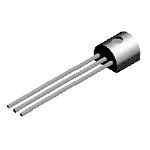General Purpose Transistor PNP
×1
 Texas Instruments 3.0V Voltage Reference
×1
 8-to-1 Multiplexer
×1
 General-Purpose Op-Amp
×1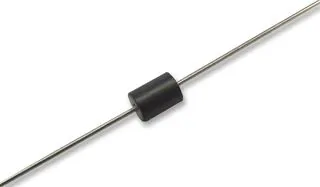×1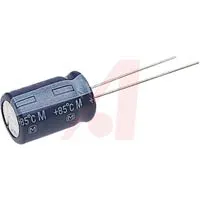Capacitor 2.2 µF
×1Capacitor 10 µF
×1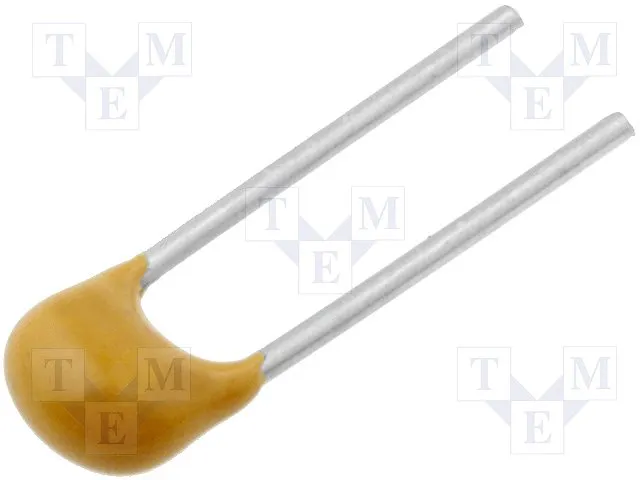Capacitor 100 nF
×5Resistor 10k ohm
×1Resistor 1k ohm
×4

### Software apps and online services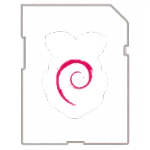Raspberry Pi RaspbianTexas Instruments Code Composer Studio
 Texas Instruments Sensor Controller Studio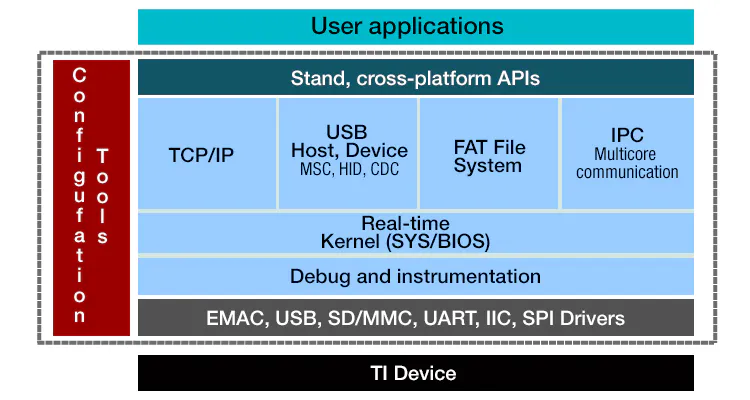Texas Instruments TI-RTOS
 Altium Designer
 Thonny

### Hand tools and fabrication machinesSoldering iron (generic)
 Infrared IC Heater

## Schematics

### PCB Layout

Layout of the printed circuit board used in this project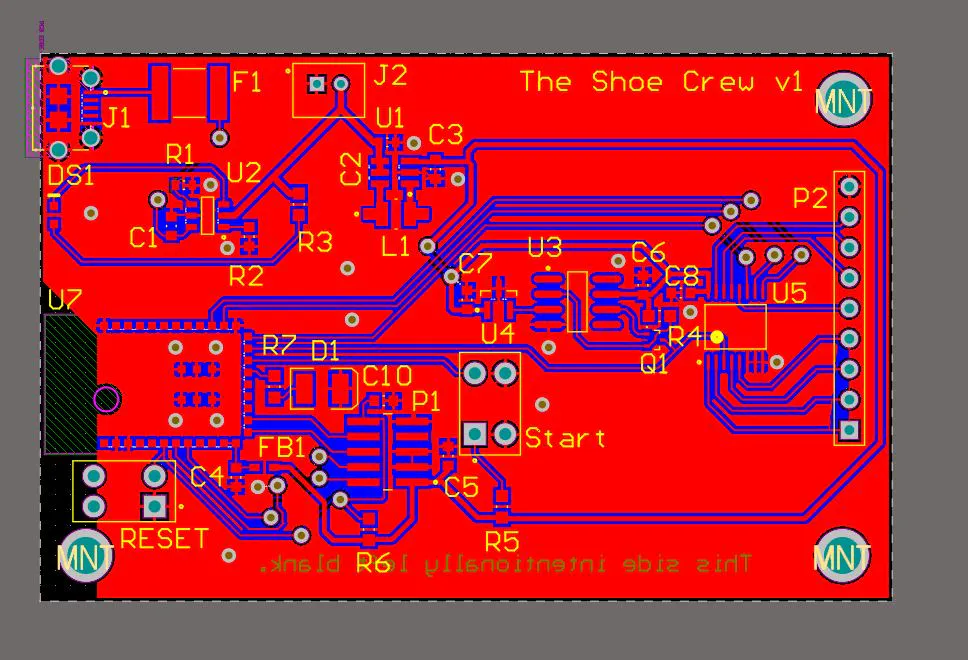### Microcontroller Schematic Document

Altium schematic document for microcontroller subsystem

### Power Supply Schematic Document

Altium schematic document for power supply subsystem

### Sensor schematic document

Altium schematic document for sensor subsystem. Connects to homemade conductive foam sensor

### PCB Library

Altium PCB Library file

### Microcontroller Schematic Image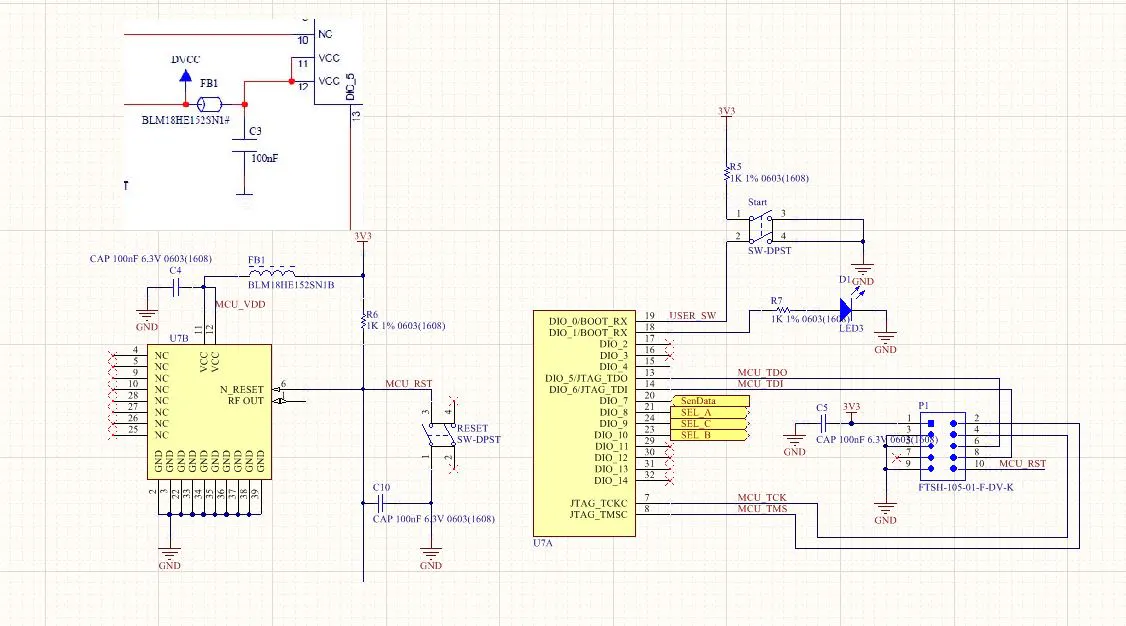### Power Supply Schematic Image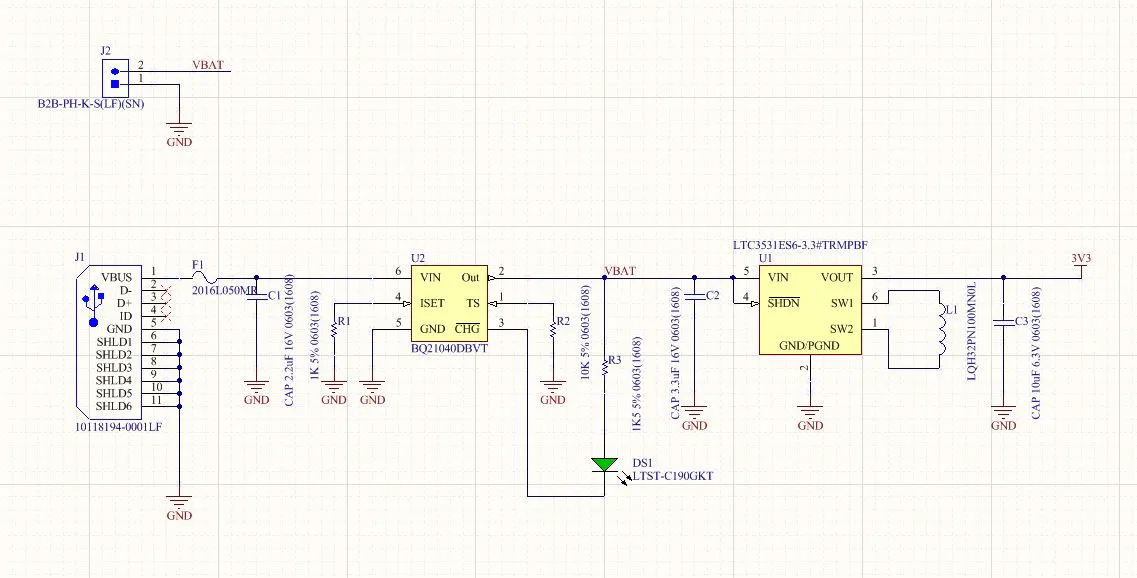### Sensor Schematic Image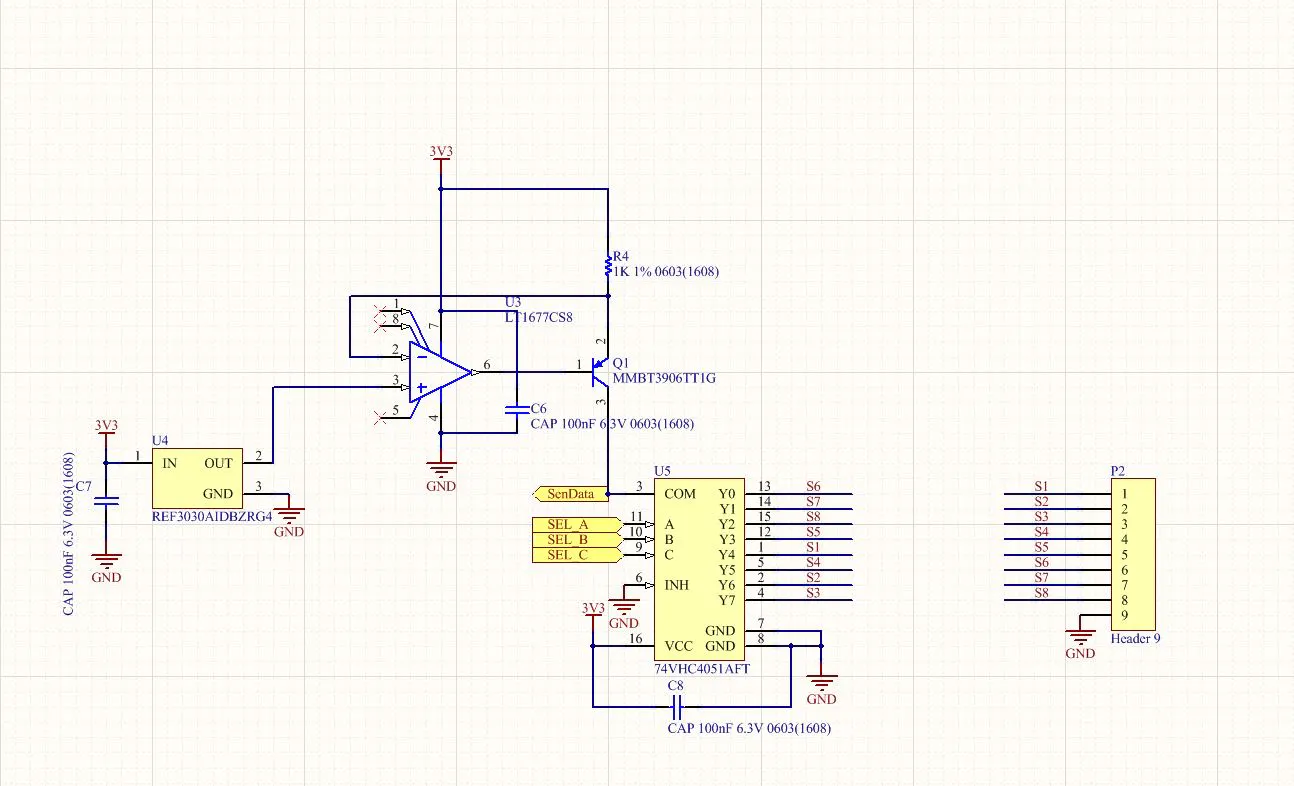## Code

### BLE Peripheral

C/C++
(This is a .zip file. Download to view) Microcontroller code for the CC2640R2 RHB based on TI's Simple Peripheral example for SDK 1_35_00_33. Reads analog data from the sensors and transmits the data to an external device (Raspberry Pi) via Bluetooth Low energy
`No preview (download only).`

### FinalProject.py

Python
Code that runs on an external Raspberry Pi. Connects to the sensor device via BLE, reads sensor data and creates a color map that updates periodically
```import matplotlib
import numpy as np
import matplotlib.pyplot as plt
import matplotlib as mpl
import matplotlib.image as mpimg
import matplotlib.cm
import pprint
import matplotlib.animation as anim
import time
import pylab
from matplotlib import colors as mcolors
from matplotlib import cm
from bluepy import btle
from matplotlib.pyplot import *

cmap = matplotlib.cm.get_cmap("jet")

print ("Connecting..")
dev = btle.Peripheral("A4:34:F1:7D:7A:E1") #PCB = A4:34:F1:7D:7A:E1, Launchpad = 54:6C:0E:A0:4D:48

print ("Services..")
svc = dev.getServiceByUUID(0xfff0);
print (str(svc))
test_data = [20, 20, 500, 2500, 1400, 1200, 2830, 34]
plot_colors = ['bo', 'bo', 'bo', 'bo', 'bo', 'bo', 'bo', 'bo']
result = [0, 0, 0, 0, 0, 0, 0, 0]

plt.ion()
fig, ax = plt.subplots()

im = ax.imshow(img)

def demo():
cl = svc.getCharacteristics(0xfff1);
for c in cl:
print (str(c))
print (data)

for b in [0,2,4,6,8,10,12,14]:
lsB = int(data[b])
msB = int(data[b+1])
r = int(b/2)
result[r] = 256*msB + lsB
print(result[r])
for q in range(8):
t_data = result[q]
if t_data < 1000:
plot_colors[q] = 'ro'
elif t_data < 2000:
plot_colors[q] = 'yo'
else:
plot_colors[q] = 'bo'

plt.plot(127,230, plot_colors, markersize=28);
plt.plot(127,200, plot_colors, markersize=28);
plt.plot(127,170, plot_colors, markersize=28);
plt.plot(145,105, plot_colors, markersize=28);
plt.plot(145,140, plot_colors, markersize=28);
plt.plot(115,140, plot_colors, markersize=28);
plt.plot(115,105, plot_colors, markersize=28);
plt.plot(110,72, plot_colors, markersize=28);

while 1:
demo()
plt.pause(0.1)
plt.draw()

dev.disconnect()
```

## Credits

### The Shoe Crew

1 project • 0 followers
A team of 4 senior ECE students from Purdue University Eashvar Venkatraman Remy Hoang Daniel Gilmore James Gradowski